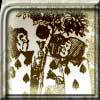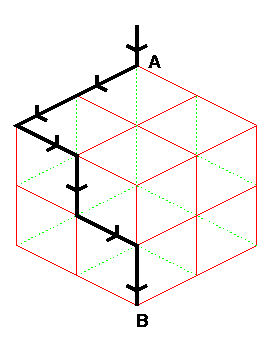#### You may also like### Card Trick 2

Can you explain how this card trick works?### Painting Cubes

Imagine you have six different colours of paint. You paint a cube using a different colour for each of the six faces. How many different cubes can be painted using the same set of six colours?### How Many Dice?

A standard die has the numbers 1, 2 and 3 are opposite 6, 5 and 4 respectively so that opposite faces add to 7? If you make standard dice by writing 1, 2, 3, 4, 5, 6 on blank cubes you will find there are 2 and only 2 different standard dice. Can you prove this ?

# Cube Paths

##### Age 11 to 14 Challenge Level:Chin Siang from Tao Nan School, Singapore solved this problem using a Pascal's Triangle method.

You are only allowed to move on the surface of the cube and only in the directions of the arrows shown in the sketch. (You might like to mark out a cube and put arrows on all the line segments and mark the numbers of routes for yourself before reading on).

How many ways can you get to each `junction point'? Look at the arrows that go towards that point and consider where you might have come from to reach it. To get the number of routes to that point you add up the numbers at the other ends of the segments which have arrows going towards it.

The numbers on the sketch give the total number of different routes to each point. So there are 54 routes from A to B. Can you see the

symmetry?